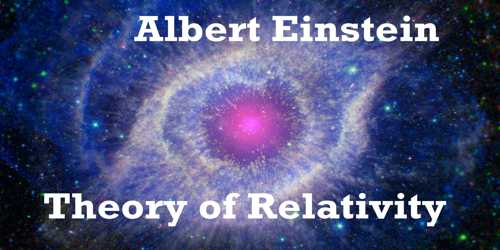# Special Theory of Relativity and its Fundamental Postulates

Special Theory of Relativity and its Fundamental Postulates

The fundamental postulates: In 1905, famous scientist Albert Einstein introduced the special theory of relativity. This special relativity is based on the following two fundamental postulates. These two postulates are called fundamental postulates of the special theory of relativity. The two postulates are stated and discussed below.

First postulate: The first law of special relativity states that the laws of physics may be expressed in equations having the same form in all frames of reference moving at constant velocity with respect to one another.

Explanation: The frame of reference in which the first law of Newton’s laws of motion is applied is called inertial frame of reference. It any object is in inertia (stationary or moving), then its state will not change unless an external force is applied to it. According to this postulate if two observers are in linear motion at constant velocity, then any physical law will remain unchanged. Any inertial reference frame is as good as any other for expressing the laws of physics because the laws are same in all such frames.

Example: A passenger of a train which is moving at constant velocity cannot prove by doing any experiment inside the compartment whether the train is at rest or in motion. Results of all experiments of physics performed in the train, whether it is at rest or in uniform motion, will be same.

Second postulate: In the vacuum, the speed of light, measured in any inertial reference frame, always has the same value of ‘c’ to all observers. This speed does not depend on the direction of transmission of light and relative velocity of the source and the observer.

Explanation: Due to this postulate the existence of ether medium cannot be accepted at all. Before Einstein scientists believed that light traveled at the speed c only when measured with respect to the ether. According to this view, an observer moving relative to the ether would measure a speed for light that was slower or faster than ‘c’, depending on whether the observer moved with or against the light. But Michelson-Morley’s experiment and later on other experiments proved that speed of light did not depend on the motion of the observer relative to the source of the light. It is a constant.